# 前端同学如何函数式编程？## 前端同学为什么要学习函数式编程思想

React框架的组件从很早开始就是不仅支持类式组件，也支持函数式的组件。 比如:下面的类继承的方式更符合大多数学过面向对象编程思想同学的心智：

``````class Welcome extends React.Component {
render() {
return <h1>Hello, {this.props.name}</h1>;
}
}
``````

``````function Welcome(props) {
return <h1>Hello, {props.name}</h1>;
}
``````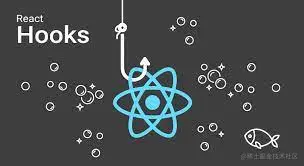``````import React, { useState } from 'react';

function Example() {
// Declare a new state variable, which we'll call "count"
const [count, setCount] = useState(0);

return (
<div>
<p>You clicked {count} times</p>
<button onClick={() => setCount(count + 1)}>
Click me
</button>
</div>
);
}
``````

``````import React, { useState, useEffect } from 'react';

function Example() {
const [count, setCount] = useState(0);

// Similar to componentDidMount and componentDidUpdate:
useEffect(() => {
// Update the document title using the browser API
document.title = `You clicked \${count} times`;
});

return (
<div>
<p>You clicked {count} times</p>
<button onClick={() => setCount(count + 1)}>
Click me
</button>
</div>
);
}
``````

Mutations, subscriptions, timers, logging, and other side effects are not allowed inside the main body of a function component (referred to as React’s render phase). Doing so will lead to confusing bugs and inconsistencies in the UI.

Instead, use `useEffect`. The function passed to `useEffect` will run after the render is committed to the screen. Think of effects as an escape hatch from React’s purely functional world into the imperative world.

``````var getRawBody = require('raw-body')
module.exports.handler = function (request, response, context) {
for (var key in reqHeader) {
};

// get request info
var url = request.url
var path = request.path
var queries = request.queries
var queryStr = ''
for (var param in queries) {
queryStr += param + "=" + queries[param] + '  '
};
var method = request.method
var clientIP = request.clientIP

// get request body
getRawBody(request, function (err, data) {
var body = data
// you can deal with your own logic here

// set response
var respBody = new Buffer('requestHeader:' + headerStr + '\n' + 'url: ' + url + '\n' + 'path: ' + path + '\n' + 'queries: ' + queryStr + '\n' + 'method: ' + method + '\n' + 'clientIP: ' + clientIP + '\n' + 'body: ' + body + '\n')
response.setStatusCode(200)
response.send(respBody)
})
};
``````

## 函数式编程的精髓：无副作用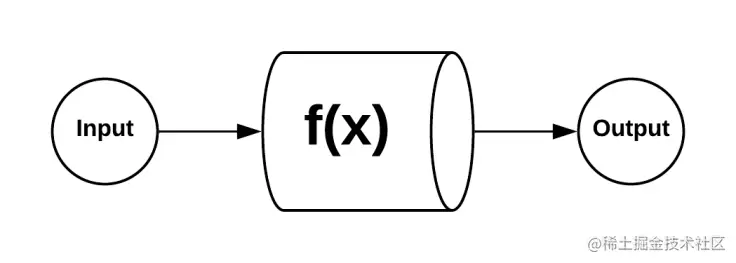1. 要有输入参数。如果没有输入参数，这个函数拿不到任意外部信息，也就不用运行了。
2. 要有返回值。如果有输入没有返回值，又没有副作用，那么这个函数白调了。
3. 对于确定的输入，有确定的输出

``````let sqr2 = function(x){
return x * x;
}
console.log(sqr2(200));
``````

1. 可以进行缓存。我们就可以采用动态规划的方法保存中间值，用来代替实际函数的执行结果，大大提升效率。
2. 可以进行高并发。因为不依赖于环境，可以调度到另一个线程、worker甚至其它机器上，反正也没有环境依赖。
3. 容易测试，容易证明正确性。不容易产生偶现问题，也跟环境无关，非常利于测试。

## 用函数的组合来代替命令的组合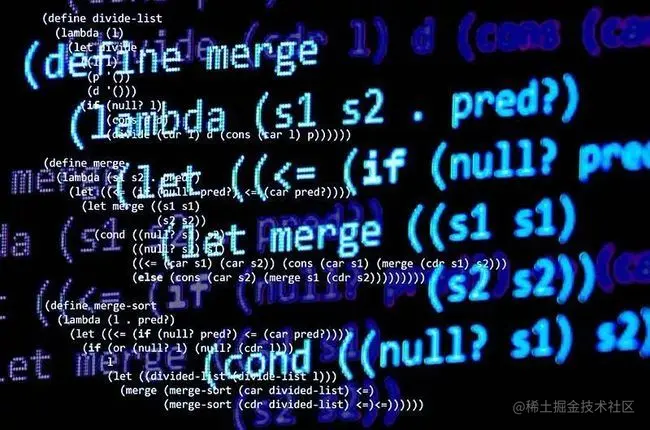``````let sqr2 = function(x){
if (typeof x === 'number'){
return x * x;
}else{
return 0;
}
}
``````

``````let isNum = function(x){
if (typeof x === 'number'){
return x;
}else{
return 0;
}
}
console.log(sqr2(isNum("20")));
``````

``````let sqr2_v3 = function(fn, x){
let y = fn(x);
return y * y;
}
console.log((sqr2_v3(isNum,1.1)));
``````

``````let sqr2_v4 = function(x){
return sqr2_v3(isNum,x);
}
console.log((sqr2_v4(2.2)));
``````

## 用容器封装函数能力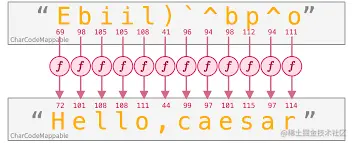``````console.log(Math.sin(undefined));
``````

``````class MayBeNumber{
constructor(x){
this.x = x;
}

map(fn){
return new MayBeNumber(fn(isNum(this.x)));
}

getValue(){
return this.x;
}
}
``````

``````let num1 = new MayBeNumber(3.3).map(sqr2).getValue();
console.log(num1);
let notnum1 = new MayBeNumber(undefined).map(sqr2).getValue();
console.log(notnum1);
``````

``````let notnum2 = new MayBeNumber(undefined).map(Math.sin).getValue();
console.log(notnum2);
``````

``````let num3 = new MayBeNumber(3.5).map(sqr2).map(sqr2).getValue();
console.log(num3);
``````

``````console.log(sqr2(Math.sin(1)));
``````

``````let num4 = new MayBeNumber(1).map(Math.sin).map(sqr2).getValue();
console.log(num4);
``````

## 用of来封装new``````MayBeNumber.of = function(x){
return new MayBeNumber(x);
}
``````

``````let num5 = MayBeNumber.of(1).map(Math.cos).getValue();
console.log(num5);
let num6 = MayBeNumber.of(2).map(Math.tan).map(Math.exp).getValue();
console.log(num6);
``````

``````isNum2 = x => typeof x === 'number';
``````

map用isNum2和of改写下：

``````    map(fn){
if (isNum2(this.x)){
return MayBeNumber.of(fn(this.x));
}else{
return MayBeNumber.of(0);
}
}
``````

``````class Result{
constructor(Ok, Err){
this.Ok = Ok;
this.Err = Err;
}

isOk(){
return this.Err === null || this.Err === undefined;
}

map(fn){
return this.isOk() ? Result.of(fn(this.Ok),this.Err) : Result.of(this.Ok, fn(this.Err));
}
}
Result.of = function(Ok, Err){
return new Result(Ok, Err);
}

console.log(Result.of(1.2,undefined).map(sqr2));
``````

``````Result { Ok: 1.44, Err: undefined }
``````

1. 有一个用于存储值的容器
2. 这个容器提供一个map函数，作用是map函数使其调用的函数可以跟容器中的值进行计算，最终返回的还是容器的对象

``````let aa1 = Array.of(1);
console.log(aa1);
console.log(aa1.map(Math.sin));
``````

## 简化对象层级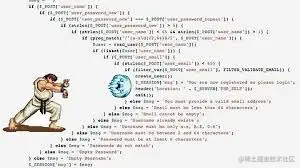``````let sqr2_Result = function(x){
if (isNum2(x)){
return Result.of(x*x, undefined);
}else{
return Result.of(undefined,0);
}
}
``````

``````console.log(Result.of(4.3,undefined).map(sqr2_Result));
``````

``````Result { Ok: Result { Ok: 18.49, Err: undefined }, Err: undefined }
``````

``````    join(){
if (this.isOk()) {
return this.Ok;
}else{
return this.Err;
}
}
``````

``````console.log(Result.of(4.5,undefined).map(sqr2_Result).join());
``````

``````Result { Ok: 20.25, Err: undefined }
``````

``````    flatMap(fn){
return this.map(fn).join();
}
``````

``````console.log(Result.of(4.7,undefined).flatMap(sqr2_Result));
``````

``````Result { Ok: 22.090000000000003, Err: undefined }
``````

``````class Result{
constructor(Ok, Err){
this.Ok = Ok;
this.Err = Err;
}

isOk(){
return this.Err === null || this.Err === undefined;
}

map(fn){
return this.isOk() ? Result.of(fn(this.Ok),this.Err) : Result.of(this.Ok, fn(this.Err));
}

join(){
if (this.isOk()) {
return this.Ok;
}else{
return this.Err;
}
}

flatMap(fn){
return this.map(fn).join();
}
}
Result.of = function(Ok, Err){
return new Result(Ok, Err);
}
``````

## 偏函数和高阶函数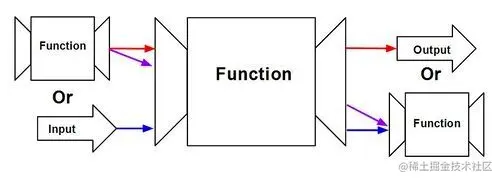1. 函数是一等公式，我们应该熟悉变量中保存函数再对其进行调用
2. 函数可以出现在返回值里，最重要的用法就是把输入是n(n>2)个参数的函数转换成n个1个参数的串联调用，这就是传说中的柯里化。这种减少了参数的新函数，我们称之为偏函数
3. 函数可以用做函数的参数，这样的函数称为高阶函数

``````const getSpm = function(spm_a, spm_b){
return [spm_a, spm_b];
}

const getSpmb = function(spm_b){
return getSpm(1000, spm_b);
}

console.log(getSpmb(1007));
``````once是一个高阶函数，返回值是一个函数，如果done是false，则将done设为true，然后执行fn。done是在返回函数的同一层，所以会被闭包记忆获取到：

``````const once = (fn) => {
let done = false;
return function() {
return done ? undefined : ((done=true), fn.apply(this,arguments));
}
}

let init_data = once(
() => {
console.log("Initialize data");
}
);

init_data();
init_data();
``````

## 递归与记忆

``````let factorial = (n) => {
if (n===0){
return 1;
}
return n*factorial(n-1);
}

console.log(factorial(10));
``````

``````const memo = (fn) => {
const cache = {};
return (arg) => cache[arg] || (cache[arg] = fn(arg));
}
``````

逻辑很简单，返回值是lamdba表达式，它仍然支持闭包，所以我们在其同层定义一个cache，然后如果cache中的某项为空则计算并保存之，如果已经有了就直接使用。

``````let fastFact = memo(
(n) => {
if (n<=0){
return 1;
}else{
return n * fastFact(n-1);
}
}
);
``````

``````const memoizedValue = useMemo(() => computeExpensiveValue(a, b), [a, b]);
``````

## 小结

1. 函数式编程的核心概念很简单，就是将函数存到变量里，用在参数里，用在返回值里
2. 在编程时要时刻记住将无副作用与有副作用代码分开
3. 函数式编程的原理虽然很简单，但是因为大家习惯了命令式编程，刚开始学习时会有诸多不习惯，用多了就好了
4. 函数式编程背后有其数学基础，在学习时可以先不要管它，当成设计模式学习。等将来熟悉之后，还是建议去了解下背后的真正原理
•••••••••••